power supply filter. The application of the simple capacitor filter is very limited. It is sometimes used on extremely high-voltage, low-current power supplies for cathode-ray and similar electron tubes, which require very little load current from the supply. The capacitor filter is also used where the power-supply ripple frequency is not critical; this frequency can be relatively high.">Custom SearchThe Capacitor Filter The simple capacitor filter is the most basic type of power supply filter. The application of the simple capacitor filter is very limited. It is sometimes used on extremely high-voltage, low-current power supplies for cathode-ray and similar electron tubes, which require very little load current from the supply. The capacitor filter is also used where the power-supply ripple frequency is not critical; this frequency can be relatively high. The capacitor (C1) shown in figure 4-15 is a simple filter connected across the output of the rectifier in parallel with the load. Figure 4-15. - Full-wave rectifier with a capacitor filter.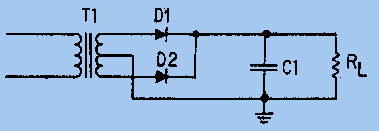When this filter is used, the RC charge time of the filter capacitor (C1) must be short and the RC discharge time must be long to eliminate ripple action. In other words, the capacitor must charge up fast, preferably with no discharge at all. Better filtering also results when the input frequency is high; therefore, the full-wave rectifier output is easier to filter than that of the half-wave rectifier because of its higher frequency. For you to have a better understanding of the effect that filtering has on Eavg, a comparison of a rectifier circuit with a filter and one without a filter is illustrated in views A and B of figure 4-16. The output waveforms in figure 4-16 represent the unfiltered and filtered outputs of the half-wave rectifier circuit. Current pulses flow through the load resistance (RL) each time a diode conducts. The dashed line indicates the average value of output voltage. For the half-wave rectifier, Eavg is less than half (or approximately 0.318) of the peak output voltage. This value is still much less than that of the applied voltage. With no capacitor connected across the output of the rectifier circuit, the waveform in view A has a large pulsating component (ripple) compared with the average or dc component. When a capacitor is connected across the output (view B), the average value of output voltage (Eavg) is increased due to the filtering action of capacitor C1. Figure 4-16A. - Half-wave rectifier with and without filtering. UNFILTERED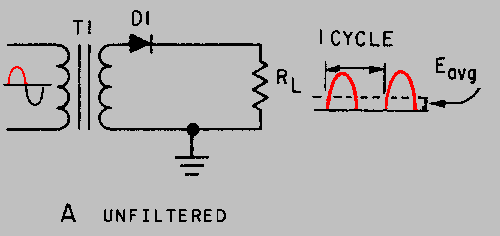Figure 4-16B. - Half-wave rectifier with and without filtering. FILTERED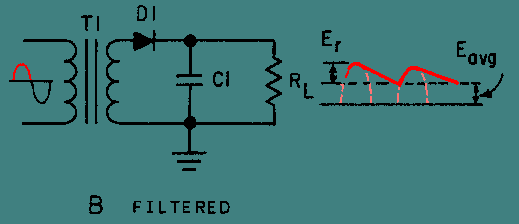The value of the capacitor is fairly large (several microfarads), thus it presents a relatively low reactance to the pulsating current and it stores a substantial charge. The rate of charge for the capacitor is limited only by the resistance of the conducting diode which is relatively low. Therefore, the RC charge time of the circuit is relatively short. As a result, when the pulsating voltage is first applied to the circuit, the capacitor charges rapidly and almost reaches the peak value of the rectified voltage within the first few cycles. The capacitor attempts to charge to the peak value of the rectified voltage anytime a diode is conducting, and tends to retain its charge when the rectifier output falls to zero. (The capacitor cannot discharge immediately.) The capacitor slowly discharges through the load resistance (RL) during the time the rectifier is nonconducting. The rate of discharge of the capacitor is determined by the value of capacitance and the value of the load resistance. If the capacitance and load-resistance values are large, the RC discharge time for the circuit is relatively long. A comparison of the waveforms shown in figure 4-16 (view A and view B) illustrates that the addition of C1 to the circuit results in an increase in the average of the output voltage (Eavg) and a reduction in the amplitude of the ripple component (Er) which is normally present across the load resistance. Now, let's consider a complete cycle of operation using a half-wave rectifier, a capacitive filter (C1), and a load resistor (RL). As shown in view A of figure 4-17, the capacitive filter (C1) is assumed to be large enough to ensure a small reactance to the pulsating rectified current. The resistance of RL is assumed to be much greater than the reactance of C1 at the input frequency. When the circuit is energized, the diode conducts on the positive half cycle and current flows through the circuit, allowing C1 to charge. C1 will charge to approximately the peak value of the input voltage. (The charge is less than the peak value because of the voltage drop across the diode (D1)). In view A of the figure, the charge on C1 is indicated by the heavy solid line on the waveform. As illustrated in view B, the diode cannot conduct on the negative half cycle because the anode of D1 is negative with respect to the cathode. During this interval, C1 discharges through the load resistor (RL). The discharge of C1 produces the downward slope as indicated by the solid line on the waveform in view B. In contrast to the abrupt fall of the applied ac voltage from peak value to zero, the voltage across C1 (and thus across RL) during the discharge period gradually decreases until the time of the next half cycle of rectifier operation. Keep in mind that for good filtering, the filter capacitor should charge up as fast as possible and discharge as little as possible. Figure 4-17A. - Capacitor filter circuit (positive and negative half cycles). POSITIVE HALF-CYCLE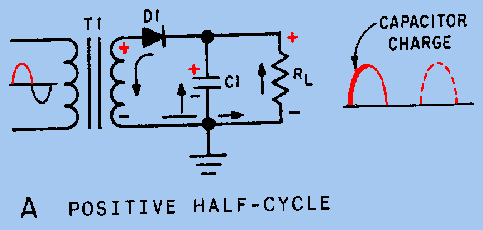Figure 4-17B. - Capacitor filter circuit (positive and negative half cycles). NEGATIVE HALF-CYCLE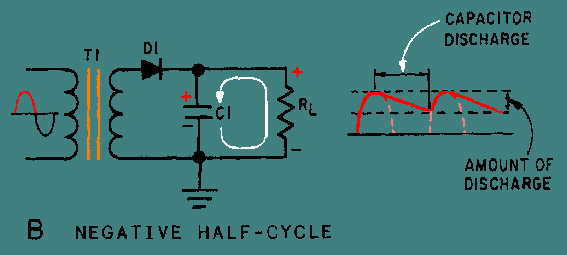Since practical values of C1 and RL ensure a more or less gradual decrease of the discharge voltage, a substantial charge remains on the capacitor at the time of the next half cycle of operation. As a result, no current can flow through the diode until the rising ac input voltage at the anode of the diode exceeds the voltage on the charge remaining on C1. The charge on C1 is the cathode potential of the diode. When the potential on the anode exceeds the potential on the cathode (the charge on C1), the diode again conducts, and C1 begins to charge to approximately the peak value of the applied voltage. After the capacitor has charged to its peak value, the diode will cut off and the capacitor will start to discharge. Since the fall of the ac input voltage on the anode is considerably more rapid than the decrease on the capacitor voltage, the cathode quickly become more positive than the anode, and the diode ceases to conduct. Operation of the simple capacitor filter using a full-wave rectifier is basically the same as that discussed for the half-wave rectifier. Referring to figure 4-18, you should notice that because one of the diodes is always conducting on. either alternation, the filter capacitor charges and discharges during each half cycle. (Note that each diode conducts only for that portion of time when the peak secondary voltage is greater than the charge across the capacitor.) Figure 4-18. - Full-wave rectifier (with capacitor filter).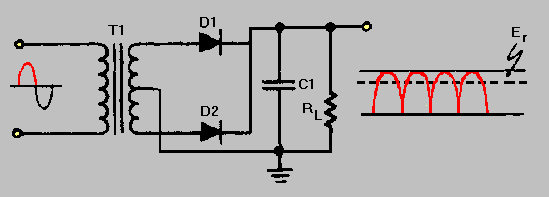Another thing to keep in mind is that the ripple component (E r) of the output voltage is an ac voltage and the average output voltage (Eavg) is the dc component of the output. Since the filter capacitor offers a relatively low impedance to ac, the majority of the ac component flows through the filter capacitor. The ac component is therefore bypassed (shunted) around the load resistance, and the entire dc component (or Eavg) flows through the load resistance. This statement can be clarified by using the formula for XC in a half-wave and full-wave rectifier. First, you must establish some values for the circuit.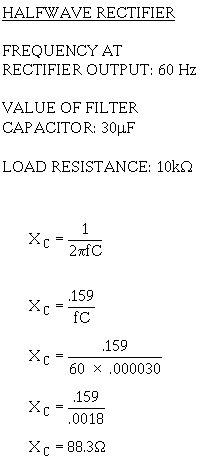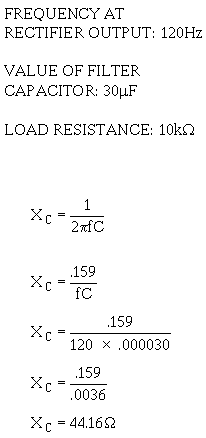As you can see from the calculations, by doubling the frequency of the rectifier, you reduce the impedance of the capacitor by one-half. This allows the ac component to pass through the capacitor more easily. As a result, a full-wave rectifier output is much easier to filter than that of a half-wave rectifier. Remember, the smaller the XC of the filter capacitor with respect to the load resistance, the better the filtering action. Since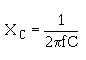the largest possible capacitor will provide the best filtering. Remember, also, that the load resistance is an important consideration. If load resistance is made small, the load current increases, and the average value of output voltage (Eavg) decreases. The RC discharge time constant is a direct function of the value of the load resistance; therefore, the rate of capacitor voltage discharge is a direct function of the current through the load. The greater the load current, the more rapid the discharge of the capacitor, and the lower the average value of output voltage. For this reason, the simple capacitive filter is seldom used with rectifier circuits that must supply a relatively large load current. Using the simple capacitive filter in conjunction with a full-wave or bridge rectifier provides improved filtering because the increased ripple frequency decreases the capacitive reactance of the filter capacitor. Q.14 What is the most basic type of filter?Q.15 In a capacitor filter, is the capacitor in series or in parallel with the load?Q.16 Is filtering better at a high frequency or at a low frequency?Q.17 Does a filter circuit increase or decrease the average output voltage?Q.18 What determines the rate of discharge of the capacitor in a filter circuit?Q.19 Does low ripple voltage indicate good or bad filtering?Q.20 Is a full-wave rectifier output easier to filter than that of a half-wave rectifier?